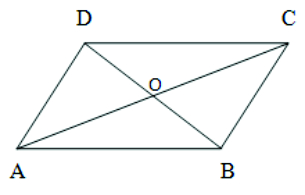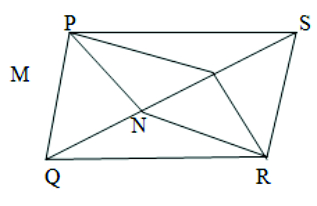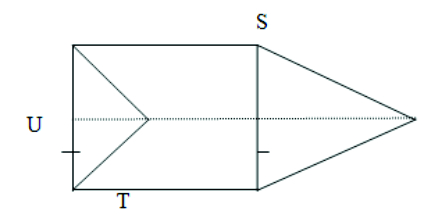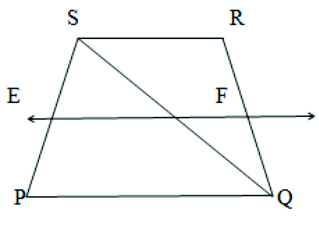8.7  TEST YOURSELF:

1. Show that the diagonals of a rhombus are perpendicular to each other.1. Show that the diagonals of a square are equal and bisect each other at right angles.

1. In parallelogram PQRS, two points M and N Pare taken on diagonal QS such that QN = MS.Show that : (i)  PMS  ≅  RNQ

(ii) PM = RN

(iii) PNQ  ≅  RMS

1. ABCD is a rhombus. Show that diagonal AC bisects ∠A as well as ∠C.

1. In PQR and STU, PQ = ST, PQ  ST= QR = UT and QR = UT.  Vertices P, Q and R are joined to vertices P, Q and R respectively.Show that (i) quadrilateral PQTS is a parallelogram.

(ii) quadrilateral PQTS is a parallelogram

(iii) PQR ≅STU

1. PQRS is a rhombus and A,B,C,D are the mid-point of the sides PQ, QR, RS, PS respectively. Show that the quadrilateral ABCD is a rectangle.

1. PQRS is a trapezium in which PQ RS,QS is the diagonal and E is the mid-point of PS.

A line is drawn through E parallel to PQ intersects QR at F.  Show that F is the mid-point of QR.